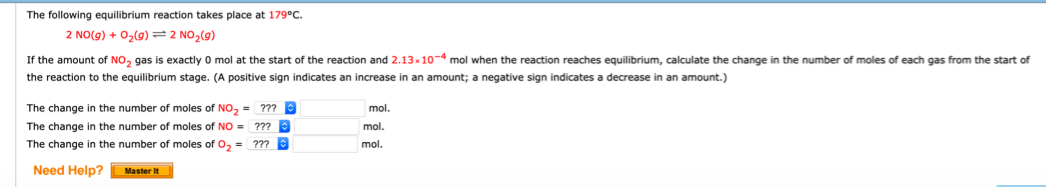# The following equilibrium reaction takes place at79°C. 2 NO(g) + 02(g) -=" 2 NO2(g) If the amount of NO2 gas is exactly 0 mol at the start of the reaction and 2.13 h 107 mol when the reaction reaches equilibrium, calculate the change in the number of moles of each gas from the start of the reaction to the equilibrium stage. (A positive sign indicates an increase in an amount; a negative sign indicates a decrease in an amount.) The change in the number of moles of NO2 = ???mol. The change in the number of moles of NO = ???mol The change in the number of moles of 02 = ???mol